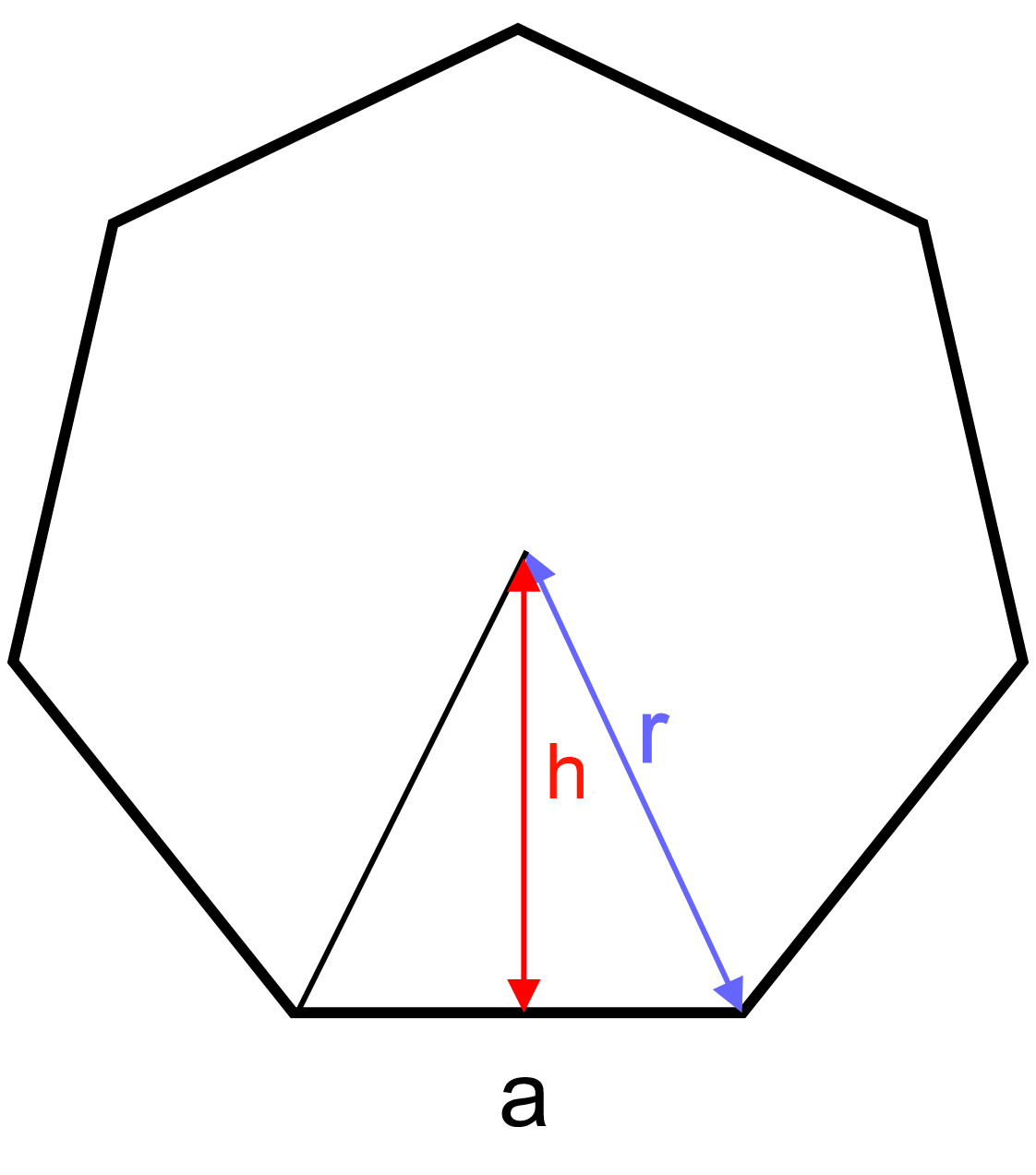﻿ Polygon: - on line calculation, formula - FORMIAX

# Polygon - circumference and surfaceC = n * a

S = (na 2)/4 * cot(π/n)

C = circumference

S = surface area

a = side

h = height

n= number of sides

WHAT IS IT ?

Polygon is a plane shape (two-dimensional) with straight sides. Examples: triangles, rectangles and pentagons.

CALCULATION:

Enter unit e.g.: inch

Enter side "a"

Enter number of sides

Round to number of decimals places

YOU MIGHT BE INTERESTED: